# Knot (mathematics)

Knot (mathematics)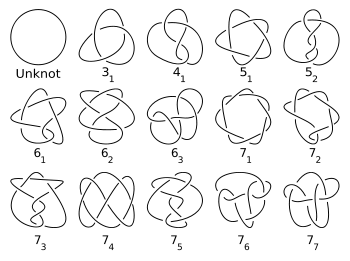A table of all prime knots with seven crossings or fewer (not including mirror images).

In mathematics, a knot is an embedding of a circle in 3-dimensional Euclidean space, R3, considered up to continuous deformations (isotopies). A crucial difference between the standard mathematical and conventional notions of a knot is that mathematical knots are closed—there are no ends to tie or untie on a mathematical knot. Physical properties such as friction and thickness also do not apply, although there are mathematical definitions of a knot that take such properties into account. The term knot is also applied to embeddings of Sj in Sn, especially in the case j = n − 2. The branch of mathematics that studies knots is known as knot theory.

## Types of knots

The simplest knot, called the unknot, is a round circle embedded in R3. In the ordinary sense of the word, the unknot is not "knotted" at all. The simplest nontrivial knots are the trefoil knot (31 in the table), the figure-eight knot (41) and the cinquefoil knot (51).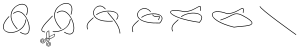A knot can be untied if the loop is broken.

Several knots, possibly tangled together, are called links. Knots are links with a single component.

Often mathematicians prefer to consider knots embedded into the 3-sphere, S3, rather than R3 since the 3-sphere is compact. The 3-sphere is equivalent to R3 with a single point added at infinity (see one-point compactification).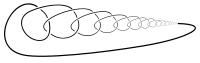A wild knot.

A knot is tame if it can be "thickened up", that is, if there exists an extension to an embedding of the solid torus,$S^1 \times D^2$, into the 3-sphere. A knot is tame if and only if it can be represented as a finite closed polygonal chain. Knots that are not tame are called wild and can have pathological behavior. In knot theory and 3-manifold theory, often the adjective "tame" is omitted. Smooth knots, for example, are always tame.

Given a knot in the 3-sphere, the knot complement is all the points of the 3-sphere not contained in the knot. A major theorem of Gordon and Luecke states that at most two knots have homeomorphic complements (the original knot and its mirror reflection). This in effect turns the study of knots into the study of their complements, and in turn into 3-manifold theory.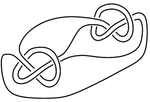A knot whose complement has a non-trivial JSJ decomposition.

The JSJ decomposition and Thurston's hyperbolization theorem reduces the study of knots in the 3-sphere to the study of various geometric manifolds via splicing or satellite operations. In the pictured knot, the JSJ-decomposition splits the complement into the union of three manifolds: two trefoil complements and the complement of the Borromean rings. The trefoil complement has the geometry of$H^2 \times R$, while the Borromean rings complement has the geometry of H3.

## Knots, more generally speaking

In contemporary mathematics the term knot is sometimes used to describe a more general phenomenon related to embeddings. Given a manifold M with a submanifold N, one sometimes says N can be knotted in M if there exists an embedding of N in M which is not isotopic to N. Traditional knots form the case where N = S1 and$M=\mathbb R^3$ or M = S3.

The Schoenflies theorem states that the circle does not knot in the 2-sphere -- every circle in the 2-sphere is isotopic to the standard circle. Alexander's theorem states that the 2-sphere does not smoothly (or PL or tame topologically) knot in the 3-sphere. In the tame topological category, it's known that the n-sphere does not knot in the n + 1-sphere for all n. This is a theorem of Brown and Mazur. The Alexander horned sphere is an example of a knotted 2-sphere in the 3-sphere which is not tame. In the smooth category, the n-sphere is known not to knot in the n + 1-sphere provided$n \neq 3$. The case n = 3 is a long-outstanding problem closely related to the question: does the 4-ball admit an exotic smooth structure?

Haefliger proved that there are no smooth j-dimensional knots in Sn provided 2n − 3j − 3 > 0, and gave further examples of knotted spheres for all$n > j \geq 1$ such that 2n − 3j − 3 = 0. nj is called the codimension of the knot. An interesting aspect of Haefliger's work is that the isotopy classes of embeddings of Sj in Sn form a group, with group operation given by the connect sum, provided the co-dimension is greater than two. Haefliger based his work on Smale's h-cobordism theorem. One of Smale's theorems is that when one deals with knots in co-dimension greater than two, even inequivalent knots have diffeomorphic complements. This gives the subject a different flavour than co-dimension 2 knot theory. If one allows topological or PL-isotopies, Zeeman proved that spheres do not knot when the co-dimension is larger than two. See a generalization to manifolds.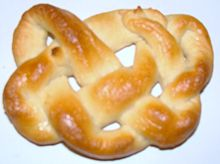• David W. Farmer & Theodore B. Stanford, Knots and Surfaces: A Guide to Discovering Mathematics, 1995.
• Colin C. Adams, The Knot Book: An Elementary Introduction to the Mathematical Theory of Knots, W. H. Freeman & Company, March 1994.
• Charles Livingstone, Knot Theory, The Mathematical Association of America, September 1996.

Wikimedia Foundation. 2010.

### Look at other dictionaries:

• Figure-eight knot (mathematics) — In knot theory, a figure eight knot (also called Listing s knot) is the unique knot with a crossing number of four. This is the smallest possible crossing number except for the unknot and trefoil knot. Origin of name The name is given because… …   Wikipedia

• Knot theory — A three dimensional depiction of a thickened trefoil knot, the simplest non trivial knot …   Wikipedia

• Knot — For speeds, see Knot (unit). For other uses, see Knot (disambiguation). Some knots 1 Yarn knot ABoK #2688 2 Manrope knot 3 Granny knot 4 Rosebud stopper knot(?) …   Wikipedia

• Knot tabulation — Ever since Sir William Thomson s vortex theory, mathematicians have tired to classify and tabulate all possible knots. As of May 2008 all prime knots up to 16 crossings have been tabulated.BeginningsIn an attempt to make a periodic table of the… …   Wikipedia

• Mathematics and art — have a long historical relationship. The ancient Egyptians and ancient Greeks knew about the golden ratio, regarded as an aesthetically pleasing ratio, and incorporated it into the design of monuments including the Great Pyramid, the Parthenon …   Wikipedia

• knot theory — ▪ mathematics       in mathematics, the study of closed curves in three dimensions, and their possible deformations without one part cutting through another. Knots may be regarded as formed by interlacing and looping a piece of string in any… …   Universalium

• List of knot theory topics — Knot theory is the study of mathematical knots. While inspired by knots which appear in daily life in shoelaces and rope, a mathematician s knot differs in that the ends are joined together so that it cannot be undone. In precise mathematical… …   Wikipedia

• Knot invariant — In the mathematical field of knot theory, a knot invariant is a quantity (in a broad sense) defined for each knot which is the same for equivalent knots. The equivalence is often given by ambient isotopy but can be given by homeomorphism. Some… …   Wikipedia

• Figure-of-eight knot — knot details | name=Figure of eight knot names=Figure eight knot, Savoy knot, Flemish knot, double stopper type=stopper strength=80% origin=Ancient related=Stevedore knot, Figure of eight loop, Figure of eight follow through, Directional figure… …   Wikipedia

• Knot group — In mathematics, a knot is an embedding of a circle into 3 dimensional Euclidean space. The knot group of a knot K is defined as the fundamental group of the knot complement of K in R3,:pi 1(mathbb{R}^3 ackslash K).Two equivalent knots have… …   Wikipedia